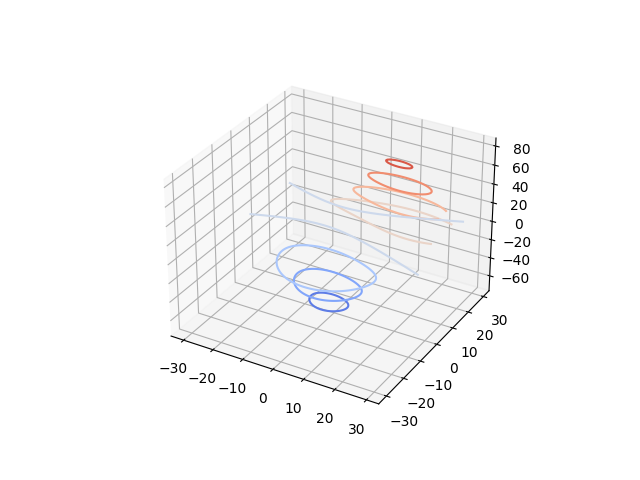# Demonstrates plotting contour (level) curves in 3D#

This is like a contour plot in 2D except that the f(x, y)=c curve is plotted on the plane z=c.from mpl_toolkits.mplot3d import axes3d
import matplotlib.pyplot as plt
from matplotlib import cm